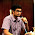## Jan 24, 2013

### Visualizing Kinect's 3D mesh with Processing

If you use Processing and SimpleOpenNI / openKinect you can visualize the mesh / surface reconstructed from Kinect's pointclouds with a simple algorithm...

The algorithm simply reconstructs the triangles taking into account that the pointcloud is organized in rows and collumns, so adjacent x-y pixels' points will be connected by triangles:

You have to mind the order of the vertex when you create the face, because changing clockwise / counterclockwise will flip the face's normals and affect the render, for example when you use lighting.

Here you can see what the normals look like as drawed by the mesh object of toxic libs (the mesh detail is reduced to show a cleaner look):

Here is the loop that draws the mesh:

for(int y=0;y < kdh-steps;y+=steps)
{
int y_steps_kdw = (y+steps)*kdw;
int y_kdw = y * kdw;
for(int x=0;x < kdw-steps;x+=steps)
{
i00 = x + y_kdw;
i01 = x + y_steps_kdw;
i10 = (x + steps) + y_kdw;
i11 = (x + steps) + y_steps_kdw;

p00 = realWorldMap[i00];
p01 = realWorldMap[i01];
p10 = realWorldMap[i10];
p11 = realWorldMap[i11];
beginShape(TRIANGLES);
texture(rgbImage); // fill the triangle with the rgb texture
if ((p00.z > 0) && (p01.z > 0) && (p10.z > 0) && // check for non valid values
(abs(p00.z-p01.z) < max_edge_len) && (abs(p10.z-p01.z) < max_edge_len)) { // check for edge length
vertex(p00.x,p00.y,p00.z, x, y); // x,y,z,u,v   position + texture reference
vertex(p01.x,p01.y,p01.z, x, y+steps);
vertex(p10.x,p10.y,p10.z, x+steps, y);
}
if ((p11.z > 0) && (p01.z > 0) && (p10.z > 0) &&
(abs(p11.z-p01.z) < 50) && (abs(p10.z-p01.z) < max_edge_len)) {
vertex(p01.x,p01.y,p01.z, x, y+steps);
vertex(p11.x,p11.y,p11.z, x+steps, y+steps);
vertex(p10.x,p10.y,p10.z, x+steps, y);
}
endShape();
}
}

As you can see triangles ar only drawn if all of their vertex are valid ( z != 0), and if the length of all edges is small enough (not to draw triangles between distant vertex).

The full pde code (for SimpleOpenNI) is available to download here, or in the repo:
https://github.com/dasaki/kinectMeshSimple

1.Thanks a lot for this. I was searching for this all over the internet. Would you happen to know of any resource for learning more about 3D reconstruction using Kinect and Processing?

2.Happy to help!

Have a look at the source code of Shiffman's Open Kinect for Processing library and also the java code of SimpleOpenNI.
Sometimes surface reconstruction from 3D clouds can become a non trivial problem, even with ordered clouds like the one provided by the Kinect sensor, specially if you want to optimize your algorithm/system. There are several approaches like Delaunay's, etc.

3.how to get the zip file? That link gets me at least 4 DOWNLOAD buttons and they all want to install executable files on my computer. Is there a link to a zip file somewhere else?

1.I've just uploaded it to a new github repo:
https://github.com/dasaki/kinectMeshSimple

2.Thank you.